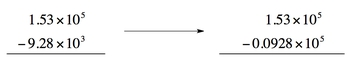Start typing, then use the up and down aroows to select an option from the list.

# GOB Chemistry

Learn the toughest concepts covered in your GOB - General, Organic, and Biological Chemistry class with step-by-step video tutorials and practice problems.

BONUS: Mathematical Operations and Functions

# Addition and Subtraction Operations

Next TopicAddition and subtraction of values in scientific notation.

## Addition and Subtraction

Whenever we add or subtract values in scientific notation we must make sure the exponents are the same value.

1

### concept

Addition and Subtraction Operations1m
Play a video:
0

If the exponents are not the same then we transform the smaller value so that they do.2

### concept

Addition and Subtraction Calculations 12m
Play a video: